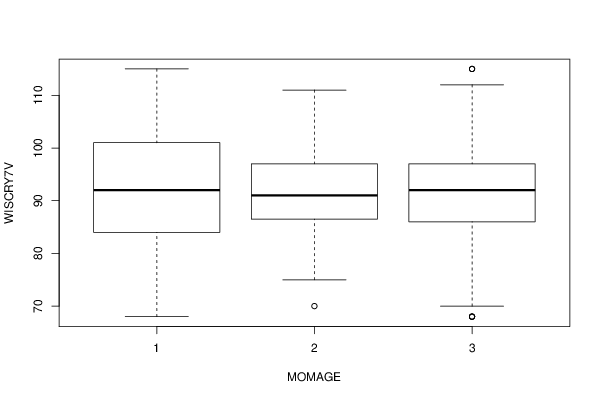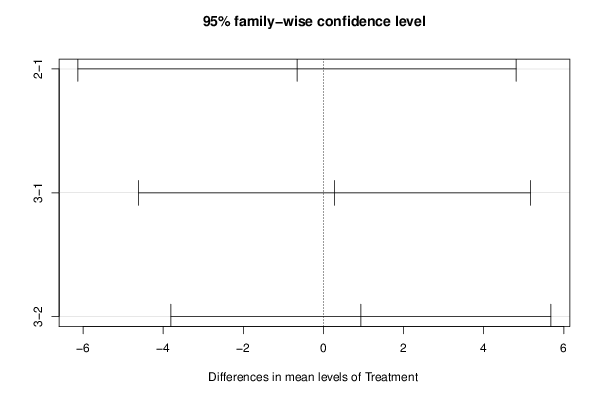## Free Statistics

of Irreproducible Research!

Author's title
Author*The author of this computation has been verified*
R Software Modulerwasp_One Factor ANOVA.wasp
Title produced by softwareOne-Way-Between-Groups ANOVA- Free Statistics Software (Calculator)
Date of computationMon, 05 Nov 2012 10:13:18 -0500
Cite this page as followsStatistical Computations at FreeStatistics.org, Office for Research Development and Education, URL https://freestatistics.org/blog/index.php?v=date/2012/Nov/05/t1352128478ikdre3cyux06awb.htm/, Retrieved Wed, 01 Feb 2023 12:52:49 +0000
Statistical Computations at FreeStatistics.org, Office for Research Development and Education, URL https://freestatistics.org/blog/index.php?pk=186100, Retrieved Wed, 01 Feb 2023 12:52:49 +0000
QR Codes:Original text written by user:
IsPrivate?No (this computation is public)
User-defined keywords
Estimated Impact71
Family? (F = Feedback message, R = changed R code, M = changed R Module, P = changed Parameters, D = changed Data)
-     [Chi Square Measure of Association- Free Statistics Software (Calculator)] [One Way ANOVA wit...] [2009-11-29 13:09:19] [98fd0e87c3eb04e0cc2efde01dbafab6]
-   PD  [Chi Square Measure of Association- Free Statistics Software (Calculator)] [One Way ANOVA for...] [2009-12-01 13:05:10] [3fdd735c61ad38cbc9b3393dc997cdb7]
- R P     [Chi Square Measure of Association- Free Statistics Software (Calculator)] [CARE date with Tu...] [2009-12-01 18:33:48] [98fd0e87c3eb04e0cc2efde01dbafab6]
-   P       [One-Way-Between-Groups ANOVA- Free Statistics Software (Calculator)] [CARE Data with Tu...] [2010-11-23 12:09:38] [3fdd735c61ad38cbc9b3393dc997cdb7]
- RM          [One-Way-Between-Groups ANOVA- Free Statistics Software (Calculator)] [IQ and Mothers Age] [2011-11-21 16:34:08] [98fd0e87c3eb04e0cc2efde01dbafab6]
- R  D            [One-Way-Between-Groups ANOVA- Free Statistics Software (Calculator)] [ANOVA - IQ at year 7] [2012-11-05 15:13:18] [596e6a605e7d57b5b185940866c8678f] [Current]
-    D              [One-Way-Between-Groups ANOVA- Free Statistics Software (Calculator)] [question 1 30 months] [2012-11-05 17:48:02] [b9554fd146071c6664c3b90882021607]
-    D                [One-Way-Between-Groups ANOVA- Free Statistics Software (Calculator)] [week 5 question 2...] [2012-11-05 17:54:14] [b9554fd146071c6664c3b90882021607]
- R PD              [One-Way-Between-Groups ANOVA- Free Statistics Software (Calculator)] [week 5 question 2...] [2012-11-05 18:44:45] [b9554fd146071c6664c3b90882021607]
- R                   [One-Way-Between-Groups ANOVA- Free Statistics Software (Calculator)] [week 5 question 2...] [2012-11-05 23:03:03] [b9554fd146071c6664c3b90882021607]
- R                   [One-Way-Between-Groups ANOVA- Free Statistics Software (Calculator)] [week 5 question 2...] [2012-11-05 23:03:03] [b9554fd146071c6664c3b90882021607]
- R PD                [One-Way-Between-Groups ANOVA- Free Statistics Software (Calculator)] [week 3 part 1] [2012-11-05 23:37:09] [b9554fd146071c6664c3b90882021607]
Feedback Forum

Post a new message
Dataseries X:
92	3
88	1
94	3
90	3
73	3
68	1
80	2
86	3
86	3
91	2
79	1
96	1
92	3
72	3
96	3
70	1
86	3
87	3
88	3
79	2
90	2
95	1
85	1
92	3
90	3
115	1
84	1
79	3
94	1
97	2
86	3
111	2
87	2
98	3
87	1
68	3
88	3
82	1
111	2
75	1
94	1
95	2
80	1
95	1
68	3
94	2
88	2
84	1
92	1
101	1
98	1
78	2
109	3
102	3
81	3
97	3
75	2
97	2
92	2
101	3
101	3
95	2
95	2
92	2
95	3
90	2
107	3
92	3
86	2
70	2
95	3
96	3
91	2
87	1
92	2
97	2
102	2
91	2
68	3
88	2
97	3
90	1
101	3
94	3
101	1
109	1
100	2
103	1
94	3
97	1
85	3
75	2
77	3
87	1
78	1
108	2
97	3
105	1
106	3
107	1
95	2
107	2
115	3
101	3
85	1
90	3
115	3
95	3
97	2
112	3
97	3
77	3
90	3
94	1
103	3
77	1
98	3
90	3
111	3
77	2
88	3
75	2
92	3
78	2
106	2
80	2
87	3
92	3
92	1
111	1
86	3
85	3
90	2
101	1
94	3
86	3
86	3
90	2
75	1
86	3
91	3
97	3
91	2
70	3
98	2
96	3
95	3
100	2
95	3
97	3
97	3
92	3
115	1
88	3
87	2
100	3
98	3
102	1
92	1
96	3


 Summary of computational transaction Raw Input view raw input (R code) Raw Output view raw output of R engine Computing time 2 seconds R Server 'Sir Maurice George Kendall' @ kendall.wessa.net R Framework error message The field 'Names of X columns' contains a hard return which cannot be interpreted. Please, resubmit your request without hard returns in the 'Names of X columns'.

\begin{tabular}{lllllllll}
\hline
Summary of computational transaction \tabularnewline
Raw Input & view raw input (R code)  \tabularnewline
Raw Output & view raw output of R engine  \tabularnewline
Computing time & 2 seconds \tabularnewline
R Server & 'Sir Maurice George Kendall' @ kendall.wessa.net \tabularnewline
R Framework error message & The field 'Names of X columns' contains a hard return which cannot be interpreted.
Please, resubmit your request without hard returns in the 'Names of X columns'. \tabularnewline
\hline
\end{tabular}
%Source: https://freestatistics.org/blog/index.php?pk=186100&T=0

[TABLE]
[ROW][C]Summary of computational transaction[/C][/ROW]
[ROW][C]Raw Input[/C][C]view raw input (R code) [/C][/ROW]
[ROW][C]Raw Output[/C][C]view raw output of R engine [/C][/ROW]
[ROW][C]Computing time[/C][C]2 seconds[/C][/ROW]
[ROW][C]R Server[/C][C]'Sir Maurice George Kendall' @ kendall.wessa.net[/C][/ROW]
[ROW][C]R Framework error message[/C][C]The field 'Names of X columns' contains a hard return which cannot be interpreted.
Please, resubmit your request without hard returns in the 'Names of X columns'.[/C][/ROW]
[/TABLE]
Source: https://freestatistics.org/blog/index.php?pk=186100&T=0

Globally Unique Identifier (entire table): ba.freestatistics.org/blog/index.php?pk=186100&T=0

As an alternative you can also use a QR Code:

The GUIDs for individual cells are displayed in the table below:

 Summary of computational transaction Raw Input view raw input (R code) Raw Output view raw output of R engine Computing time 2 seconds R Server 'Sir Maurice George Kendall' @ kendall.wessa.net R Framework error message The field 'Names of X columns' contains a hard return which cannot be interpreted. Please, resubmit your request without hard returns in the 'Names of X columns'.

 ANOVA Model WISCRY7V ~ MOMAGE means 91.75 -0.659 0.276

\begin{tabular}{lllllllll}
\hline
ANOVA Model \tabularnewline
WISCRY7V  ~  MOMAGE
\tabularnewline
means & 91.75 & -0.659 & 0.276 \tabularnewline
\hline
\end{tabular}
%Source: https://freestatistics.org/blog/index.php?pk=186100&T=1

[TABLE]
[ROW][C]ANOVA Model[/C][/ROW]
[ROW][C]WISCRY7V  ~  MOMAGE
[/C][/ROW]
[ROW][C]means[/C][C]91.75[/C][C]-0.659[/C][C]0.276[/C][/ROW]
[/TABLE]
Source: https://freestatistics.org/blog/index.php?pk=186100&T=1

Globally Unique Identifier (entire table): ba.freestatistics.org/blog/index.php?pk=186100&T=1

As an alternative you can also use a QR Code:

The GUIDs for individual cells are displayed in the table below:

 ANOVA Model WISCRY7V ~ MOMAGE means 91.75 -0.659 0.276

 ANOVA Statistics Df Sum Sq Mean Sq F value Pr(>F) MOMAGE 2 24.516 12.258 0.11 0.896 Residuals 157 17573.084 111.93

\begin{tabular}{lllllllll}
\hline
ANOVA Statistics \tabularnewline
& Df & Sum Sq & Mean Sq & F value & Pr(>F) \tabularnewline
MOMAGE
& 2 & 24.516 & 12.258 & 0.11 & 0.896 \tabularnewline
Residuals & 157 & 17573.084 & 111.93 &   &   \tabularnewline
\hline
\end{tabular}
%Source: https://freestatistics.org/blog/index.php?pk=186100&T=2

[TABLE]
[ROW][C]ANOVA Statistics[/C][/ROW]
[ROW][C] [/C][C]Df[/C][C]Sum Sq[/C][C]Mean Sq[/C][C]F value[/C][C]Pr(>F)[/C][/ROW]
[ROW][C]MOMAGE
[/C][C]2[/C][C]24.516[/C][C]12.258[/C][C]0.11[/C][C]0.896[/C][/ROW]
[ROW][C]Residuals[/C][C]157[/C][C]17573.084[/C][C]111.93[/C][C] [/C][C] [/C][/ROW]
[/TABLE]
Source: https://freestatistics.org/blog/index.php?pk=186100&T=2

Globally Unique Identifier (entire table): ba.freestatistics.org/blog/index.php?pk=186100&T=2

As an alternative you can also use a QR Code:

The GUIDs for individual cells are displayed in the table below:

 ANOVA Statistics Df Sum Sq Mean Sq F value Pr(>F) MOMAGE 2 24.516 12.258 0.11 0.896 Residuals 157 17573.084 111.93

 Tukey Honest Significant Difference Comparisons diff lwr upr p adj 2-1 -0.659 -6.128 4.81 0.956 3-1 0.276 -4.614 5.166 0.99 3-2 0.935 -3.807 5.678 0.887

\begin{tabular}{lllllllll}
\hline
Tukey Honest Significant Difference Comparisons \tabularnewline
& diff & lwr & upr & p adj \tabularnewline
2-1 & -0.659 & -6.128 & 4.81 & 0.956 \tabularnewline
3-1 & 0.276 & -4.614 & 5.166 & 0.99 \tabularnewline
3-2 & 0.935 & -3.807 & 5.678 & 0.887 \tabularnewline
\hline
\end{tabular}
%Source: https://freestatistics.org/blog/index.php?pk=186100&T=3

[TABLE]
[ROW][C]Tukey Honest Significant Difference Comparisons[/C][/ROW]
[ROW][C]2-1[/C][C]-0.659[/C][C]-6.128[/C][C]4.81[/C][C]0.956[/C][/ROW]
[ROW][C]3-1[/C][C]0.276[/C][C]-4.614[/C][C]5.166[/C][C]0.99[/C][/ROW]
[ROW][C]3-2[/C][C]0.935[/C][C]-3.807[/C][C]5.678[/C][C]0.887[/C][/ROW]
[/TABLE]
Source: https://freestatistics.org/blog/index.php?pk=186100&T=3

Globally Unique Identifier (entire table): ba.freestatistics.org/blog/index.php?pk=186100&T=3

As an alternative you can also use a QR Code:

The GUIDs for individual cells are displayed in the table below:

 Tukey Honest Significant Difference Comparisons diff lwr upr p adj 2-1 -0.659 -6.128 4.81 0.956 3-1 0.276 -4.614 5.166 0.99 3-2 0.935 -3.807 5.678 0.887

 Levenes Test for Homogeneity of Variance Df F value Pr(>F) Group 2 1.227 0.296 157

\begin{tabular}{lllllllll}
\hline
Levenes Test for Homogeneity of Variance \tabularnewline
& Df & F value & Pr(>F) \tabularnewline
Group & 2 & 1.227 & 0.296 \tabularnewline
& 157 &   &   \tabularnewline
\hline
\end{tabular}
%Source: https://freestatistics.org/blog/index.php?pk=186100&T=4

[TABLE]
[ROW][C]Levenes Test for Homogeneity of Variance[/C][/ROW]
[ROW][C] [/C][C]Df[/C][C]F value[/C][C]Pr(>F)[/C][/ROW]
[ROW][C]Group[/C][C]2[/C][C]1.227[/C][C]0.296[/C][/ROW]
[ROW][C] [/C][C]157[/C][C] [/C][C] [/C][/ROW]
[/TABLE]
Source: https://freestatistics.org/blog/index.php?pk=186100&T=4

Globally Unique Identifier (entire table): ba.freestatistics.org/blog/index.php?pk=186100&T=4

As an alternative you can also use a QR Code:

The GUIDs for individual cells are displayed in the table below:

 Levenes Test for Homogeneity of Variance Df F value Pr(>F) Group 2 1.227 0.296 157PNG link Postscript link PDF linkPNG link Postscript link PDF link

Parameters (Session):
par1 = 1 ; par2 = 2 ; par3 = TRUE ;
Parameters (R input):
par1 = 1 ; par2 = 2 ; par3 = TRUE ;
R code (references can be found in the software module):
cat1 <- as.numeric(par1) #cat2<- as.numeric(par2) #intercept<-as.logical(par3)x <- t(x)x1<-as.numeric(x[,cat1])f1<-as.character(x[,cat2])xdf<-data.frame(x1,f1)(V1<-dimnames(y)[][cat1])(V2<-dimnames(y)[][cat2])names(xdf)<-c('Response', 'Treatment')if(intercept == FALSE) (lmxdf<-lm(Response ~ Treatment - 1, data = xdf) ) else (lmxdf<-lm(Response ~ Treatment, data = xdf) )(aov.xdf<-aov(lmxdf) )(anova.xdf<-anova(lmxdf) )load(file='createtable')a<-table.start()a<-table.row.start(a)a<-table.element(a,'ANOVA Model', length(lmxdf$coefficients)+1,TRUE)a<-table.row.end(a)a<-table.row.start(a)a<-table.element(a, paste(V1, ' ~ ', V2), length(lmxdf$coefficients)+1,TRUE)a<-table.row.end(a)a<-table.row.start(a)a<-table.element(a, 'means',,TRUE)for(i in 1:length(lmxdf$coefficients)){a<-table.element(a, round(lmxdf$coefficients[i], digits=3),,FALSE)}a<-table.row.end(a)a<-table.end(a)table.save(a,file='mytable.tab')a<-table.start()a<-table.row.start(a)a<-table.element(a,'ANOVA Statistics', 5+1,TRUE)a<-table.row.end(a)a<-table.row.start(a)a<-table.element(a, ' ',,TRUE)a<-table.element(a, 'Df',,FALSE)a<-table.element(a, 'Sum Sq',,FALSE)a<-table.element(a, 'Mean Sq',,FALSE)a<-table.element(a, 'F value',,FALSE)a<-table.element(a, 'Pr(>F)',,FALSE)a<-table.row.end(a)a<-table.row.start(a)a<-table.element(a, V2,,TRUE)a<-table.element(a, anova.xdf$Df,,FALSE)a<-table.element(a, round(anova.xdf$'Sum Sq', digits=3),,FALSE)a<-table.element(a, round(anova.xdf$'Mean Sq', digits=3),,FALSE)a<-table.element(a, round(anova.xdf$'F value', digits=3),,FALSE)a<-table.element(a, round(anova.xdf$'Pr(>F)', digits=3),,FALSE)a<-table.row.end(a)a<-table.row.start(a)a<-table.element(a, 'Residuals',,TRUE)a<-table.element(a, anova.xdf$Df,,FALSE)a<-table.element(a, round(anova.xdf$'Sum Sq', digits=3),,FALSE)a<-table.element(a, round(anova.xdf$'Mean Sq', digits=3),,FALSE)a<-table.element(a, ' ',,FALSE)a<-table.element(a, ' ',,FALSE)a<-table.row.end(a)a<-table.end(a)table.save(a,file='mytable1.tab')bitmap(file='anovaplot.png')boxplot(Response ~ Treatment, data=xdf, xlab=V2, ylab=V1)dev.off()if(intercept==TRUE){thsd<-TukeyHSD(aov.xdf)bitmap(file='TukeyHSDPlot.png')plot(thsd)dev.off()}if(intercept==TRUE){a<-table.start()a<-table.row.start(a)a<-table.element(a,'Tukey Honest Significant Difference Comparisons', 5,TRUE)a<-table.row.end(a)a<-table.row.start(a)a<-table.element(a, ' ', 1, TRUE)for(i in 1:4){a<-table.element(a,colnames(thsd[])[i], 1, TRUE)}a<-table.row.end(a)for(i in 1:length(rownames(thsd[]))){a<-table.row.start(a)a<-table.element(a,rownames(thsd[])[i], 1, TRUE)for(j in 1:4){a<-table.element(a,round(thsd[][i,j], digits=3), 1, FALSE)}a<-table.row.end(a)}a<-table.end(a)table.save(a,file='mytable2.tab')}if(intercept==FALSE){a<-table.start()a<-table.row.start(a)a<-table.element(a,'TukeyHSD Message', 1,TRUE)a<-table.row.end(a)a<-table.start()a<-table.row.start(a)a<-table.element(a,'Must Include Intercept to use Tukey Test ', 1, FALSE)a<-table.row.end(a)a<-table.end(a)table.save(a,file='mytable2.tab')}library(car)lt.lmxdf<-levene.test(lmxdf)a<-table.start()a<-table.row.start(a)a<-table.element(a,'Levenes Test for Homogeneity of Variance', 4,TRUE)a<-table.row.end(a)a<-table.row.start(a)a<-table.element(a,' ', 1, TRUE)for (i in 1:3){a<-table.element(a,names(lt.lmxdf)[i], 1, FALSE)}a<-table.row.end(a)a<-table.row.start(a)a<-table.element(a,'Group', 1, TRUE)for (i in 1:3){a<-table.element(a,round(lt.lmxdf[[i]], digits=3), 1, FALSE)}a<-table.row.end(a)a<-table.row.start(a)a<-table.element(a,' ', 1, TRUE)a<-table.element(a,lt.lmxdf[], 1, FALSE)a<-table.element(a,' ', 1, FALSE)a<-table.element(a,' ', 1, FALSE)a<-table.row.end(a)a<-table.end(a)table.save(a,file='mytable3.tab')